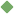newton's_second_law 1. [ noun ] (physics,mechanics) the rate of change of momentum is proportional to the imposed force and goes in the direction of the force Synonyms: Newton's_second_law_of_motion second_law_of_motion Related terms: Newton's_law_of_motion To share this definition press "text" (Facebook, Twitter) or "link" (blog, mail) then paste text link Similar spelling: Newton's_third_law   Newton's_first_law